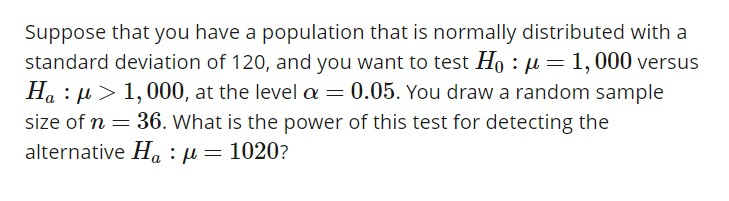# Suppose that you have a population that is normally distributed with astandard deviation of 120, and you want to test Ho : μ-1,000 versusHa : μ > 1,000, at the level α 0.05. You draw a random samplealternative Ha : μ1020?

Question
1 views

Hello. Can I get help on how to work out this problem? If there is also a way to calculate using the TI-83 graphing calculator, that would be helpful. Thanks!help_outlineImage TranscriptioncloseSuppose that you have a population that is normally distributed with a standard deviation of 120, and you want to test Ho : μ-1,000 versus Ha : μ > 1,000, at the level α 0.05. You draw a random sample alternative Ha : μ 1020? fullscreen
check_circle

star
star
star
star
star
1 Rating
Step 1

Power of a test:

Power of a test is the probability of rejecting a null hypothesis when it is false. That is, the probability of rejecting a null hypothesis when the alternative hypothesis is true. Therefore,

Step 2

The null and alternative hypotheses are H0: µ = 1,000 and Ha: µ > 1,000. A random sample of size 36 is taken from the population with standard deviation 120. The power of the test wants to calculate at the alternative Ha: µ = 1,020. The test is right tailed, therefore the critical region for the test is, Z > Za. The significance level is 0.05 and the Z- value corresponding to significance level 0.05 is 1.645. Therefore, the power of the test is given by:

Step 3

Substituting µ0 = 1,000 and σ = 120, and n=...

### Want to see the full answer?

See Solution

#### Want to see this answer and more?

Solutions are written by subject experts who are available 24/7. Questions are typically answered within 1 hour.*

See Solution
*Response times may vary by subject and question.
Tagged in

### Statistics